# Product of the sum and difference

Calculate the product of the sum and difference of numbers -7 and -2.

x =  45

### Step-by-step explanation: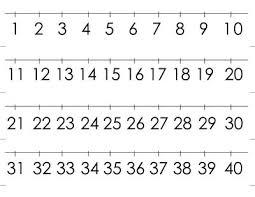Did you find an error or inaccuracy? Feel free to write us. Thank you!## Related math problems and questions:

• Integers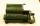May be the sum of two integers less than their difference?
• Evaluate expressionIf x=2, y=-5 and z=3 what is the value of x-2y
• Expression 6Evaluate expression: -6-2(4-8)-9
• With bracket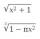Calculate (evaluate) simple mathematical expression with a negative numbers and a bracket: 13+15*5-2*(-6)
• Opposite numbers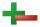Calculate opposite numbers (additive inverse) to given ones:
• Product increased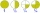When the product of 2/3 and 6/10 is increased by 2/5, the result is?
• Expression 1What is 7+8-(5×2)+5-4+(6×(5-3)+6)-(8+10)-7+6?
• MistakeNicol mistake when calculate in school. Instead of add number 20 subtract it. What is the difference between the result and the right result?
• Brackets 2Add parenthesis to make true: 5-2×6-4+2=5
• Expression plus minusEvaluate expression: (-1)2 . 12 – 6 : 3 + (-3) . (-2) + 22 – (-3) . 2
• Complicated sum minus productWhat must be subtracted from the sum of 3/8 and 5/16 to get difference equal to the product of 5/8 and 3/16?
• Evaluate - order of opsEvaluate the expression: 32+2[5×(24-6)]-48÷24 Pay attention to the order of operation including integersAdd this two mixed numbers: 1 5/6 + 2 2/11=A number decreased by the difference between four and the number.Evaluate this expressions: a) 5[3 + 4(2.8 - 3)] b) 5×(8-4)÷4-2Round the following negative number: -143.021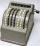Sum of the numbers 1.01 and 3.35 multiply by the difference of numbers 6.69 and 1.39.